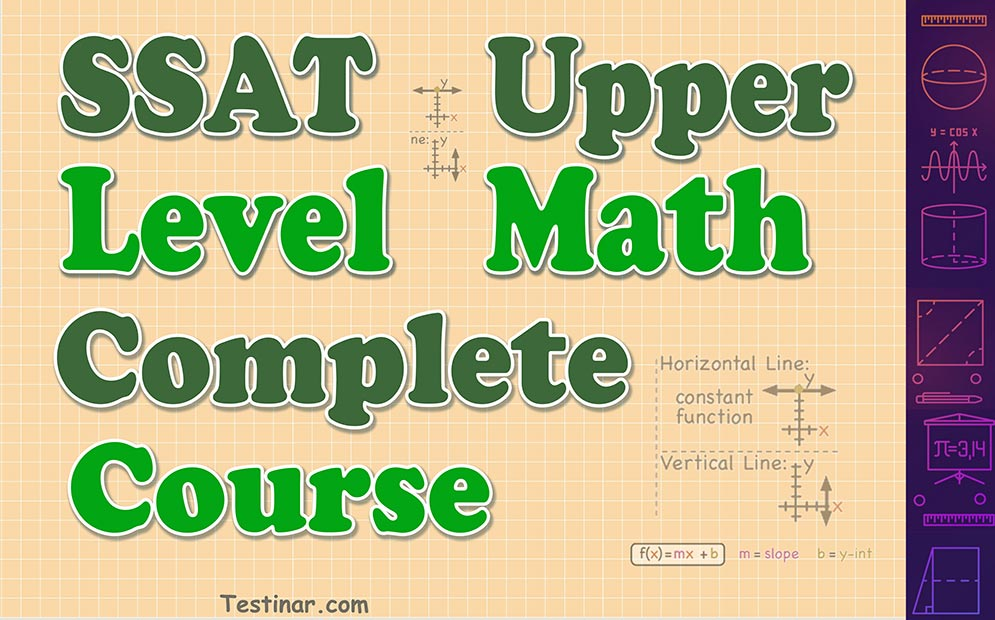## SSAT Upper-Level Math Test

The SSAT (Secondary School Admissions Test) is a standard test used to evaluate applicants for admission to private elementary, middle, and high schools.
There are currently three levels of the SSAT:

• Lower Level (for students in 3rd and 4th grade)
• Middle Level (for students in 5th-7th grade)
• Upper Level (for students in 8th-11th grade)

The SSAT Middle-Level Test includes the following segment:

1. Writing: 25 minutes.
2. Math section: 25 questions, 30 minutes.
3. Reading section: 40 questions, 40 minutes.
4. Verbal section: 60 questions, 30 minutes.
5. Math section: 25 questions, 30 minutes.
6. Experimental: 16 questions, 15 minutes.

The Upper-Level SSAT Math part covers four major Math topics:
Numbers and Operations: This section contains mathematical word problems such as percentages, estimates, rational numbers, sequences and series, and frequencies.
Algebra: assesses pupils' understanding of exponent properties, algebraic word problems, line equations, patterns, and absolute value.
Geometry and Measurement: this section analyses students' ability to calculate the area and circumference of a circle, the area and perimeter of a polygon, and the volume of a cube, cylinder, or box.
Data Analysis and Probability: analyzing data such as tables and graphs, trends and inferences, and probability is all covered.
Our expert, SSAT specialists, created a detailed FREE SSAT Upper-Level Math practice questions, worksheets, and tests to help students excel on the SSAT Upper-Level Math test. Moreover, our SSAT Math resources have been completely revised to suit the most recent SSAT modifications from 2019 to 2022.

### The Most Comprehensive SSAT Upper Level Math Preparation Bundle

\$76.99 \$36.99
52% Off*

Includes SSAT Upper Level Math Prep Books, Workbooks, and Practice Tests

### SSAT Upper Level Math for Beginners

\$24.99 \$14.99
40% Off*

The Ultimate Step by Step Guide to Preparing for the SSAT Upper Level Math Test

\$15.99 \$10.99

\$15.99 \$10.99

\$21.99 \$16.99

\$15.99 \$10.99